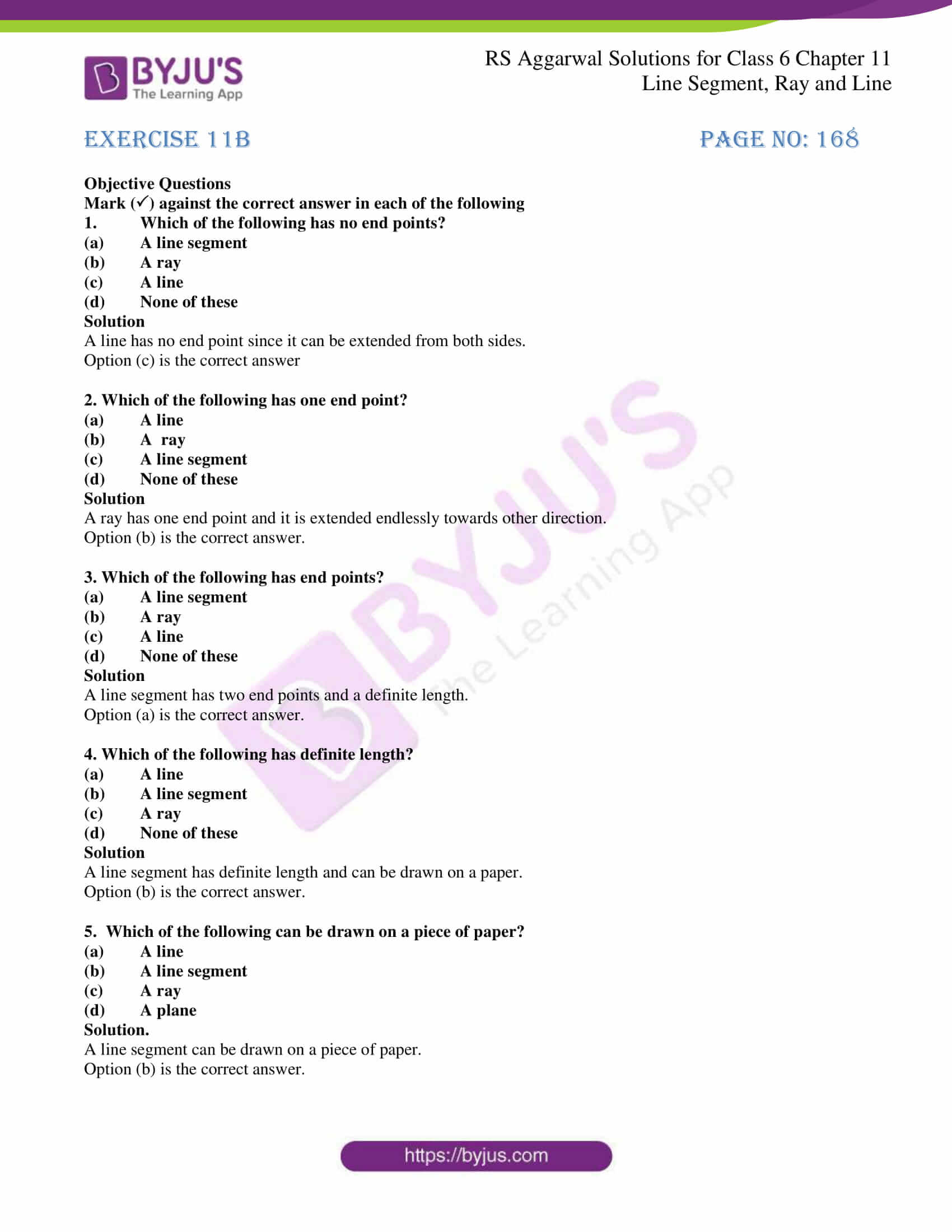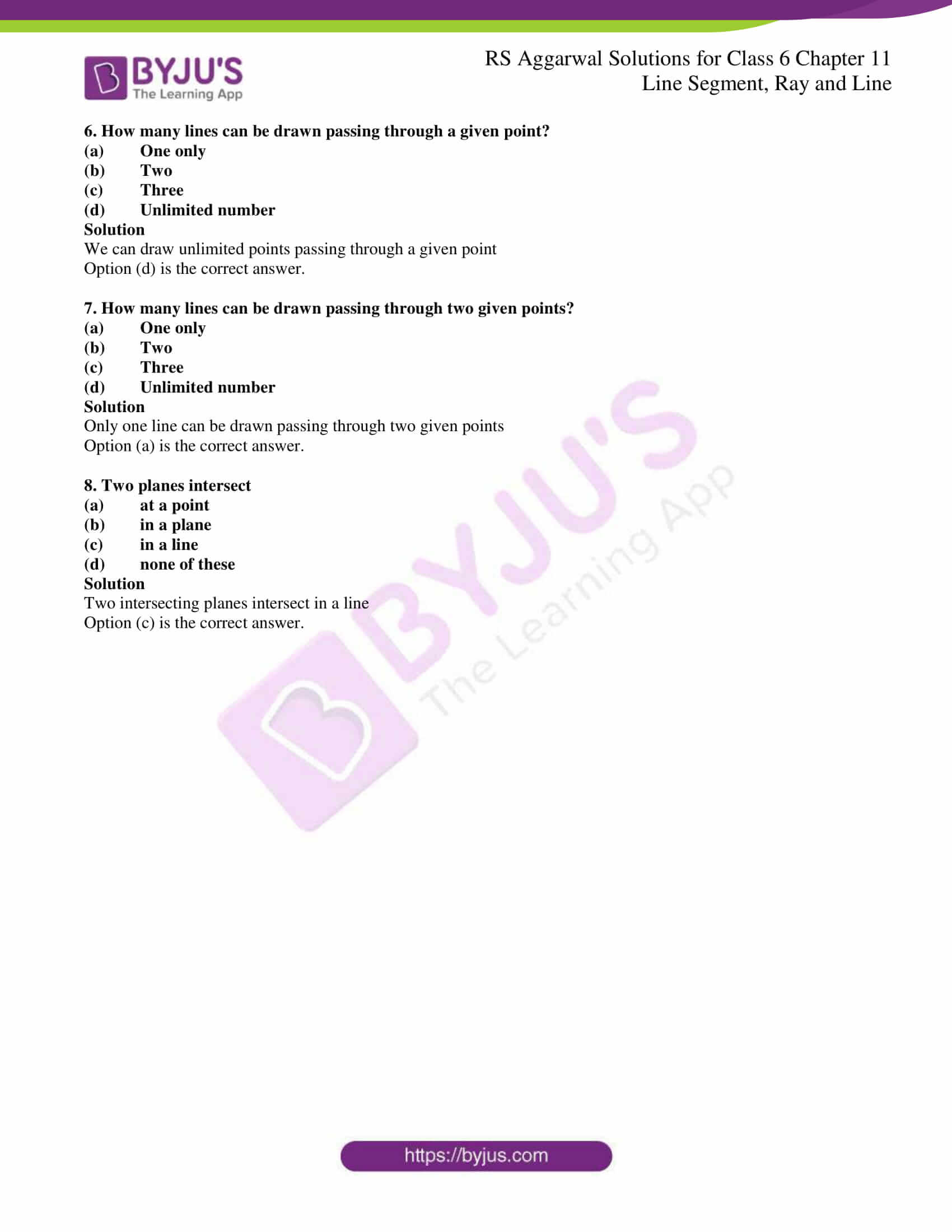# RS Aggarwal Solutions for Class 6 Chapter 11 Line Segment, Ray and Line Exercise 11B

The second exercise of chapter 11 has objective type questions which cover the entire chapter. RS Aggarwal Solutions has answers with important concepts and formulas highlighted to make them easy for the students while preparing for the exam. The accurate solutions for each problem are based on the guidelines of CBSE board. To know more about these concepts, RS Aggarwal Solutions for Class 6 Chapter 11 Line Segment, Ray and Line Exercise 11B PDF can be downloaded for free.

## Download PDF of RS Aggarwal Solutions for Class 6 Chapter 11 Line Segment, Ray and Line Exercise 11B### Access answers to Maths RS Aggarwal Solutions for Class 6 Chapter 11 Line Segment, Ray and Line Exercise 11B

Objective Questions

Mark () against the correct answer in each of the following

1. Which of the following has no end points?

(a) A line segment

(b) A ray

(c) A line

(d) None of these

Solution

A line has no end point since it can be extended from both sides.

Option (c) is the correct answer

2. Which of the following has one end point?

(a) A line

(b) A ray

(c) A line segment

(d) None of these

Solution

A ray has one end point and it is extended endlessly towards other direction.

Option (b) is the correct answer.

3. Which of the following has end points?

(a) A line segment

(b) A ray

(c) A line

(d) None of these

Solution

A line segment has two end points and a definite length.

Option (a) is the correct answer.

4. Which of the following has definite length?

(a) A line

(b) A line segment

(c) A ray

(d) None of these

Solution

A line segment has definite length and can be drawn on a paper.

Option (b) is the correct answer.

5. Which of the following can be drawn on a piece of paper?

(a) A line

(b) A line segment

(c) A ray

(d) A plane

Solution.

A line segment can be drawn on a piece of paper.

Option (b) is the correct answer.

6. How many lines can be drawn passing through a given point?

(a) One only

(b) Two

(c) Three

(d) Unlimited number

Solution

We can draw unlimited points passing through a given point

Option (d) is the correct answer.

7. How many lines can be drawn passing through two given points?

(a) One only

(b) Two

(c) Three

(d) Unlimited number

Solution

Only one line can be drawn passing through two given points

Option (a) is the correct answer.

8. Two planes intersect

(a) at a point

(b) in a plane

(c) in a line

(d) none of these

Solution

Two intersecting planes intersect in a line

Option (c) is the correct answer.

### Access other exercises of RS Aggarwal Solutions for Class 6 Chapter 11 Line Segment, Ray and Line

Exercise 11A Solutions